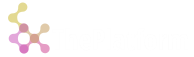# Language idioms

Language idiom is special combinations of verbs, which has better performance and memory optimizations.Idioms are recognized and optimized in lambda bodies only at parse time.

### "Type id" idiom

Syntax: `![symvec]`

where `symvec` is a constant symbol vector defining type. Idiom is calculated at parse time and replaced with actual type id as long integer. It'srecommended to use as the fastest way of checking type for strict equality.

``````o) { (!`s`long)=@x }1
1b
``````

### "Find from" idiom

Quite often it's necessary to find index of some value in list starting with some position from the left.

Syntax: `pos+(pos _ vec)?val`

where `pos` is a position to start search from, `vec` - vector to look in, `val` - value to look for.

``````o) {[vec;pos;val] pos+(pos _ vec)?val }[2 1 2 3; 1; 2]
2
``````

### "Find to" idiom

Similar to "find from" idiom, this idiom searches until some position in vector.

Syntax: `(pos#vec)?val`

where `pos` is a position to stop search, `vec` - vector to look in, `val` - value to look for.

``````o) {[vec;pos;val] (pos#vec)?val }[1 2 2 3; 2; 3]
0N
``````

### "Where equal" idiom

Optimized search of indices in `g`indexed vector. Implementation uses optimized index search if `vec` vector is long enough.

Syntax: `&vec=val` or `&val=vec`

where `vec` - vector to look in, `val` - value to look for.